# Question Assumptions

The following article written in 2006 by Mark Turner is an introduction into how Scaffold handles statistics.

Table of Contents

Statistical Assumptions

All proteomics programs that employ statistics make certain assumptions about their data sets, which can lead to untrustworthy conclusions. Doing proteomics the same way that the lab which created a given program does proteomics, then these assumptions probably hold and the statistics will probably make sense. But if you are doing something different, the underlying assumptions may not hold; this could mean wrong or missed identifications. How can you recognize when this is happening? What you can do about it?

Should You Trust the Statistics?

Scaffold validates MS/MS based protein identifications by analyzing tandem mass spectrometry data that has been processed by several search engines. It transforms the search engine scores into statistical probabilities that makes protein identifications easier to validate. This works neatly if you believe Scaffold’s statistical algorithms give correct probabilities. But do you really believe these computer algorithms? Should you? Or perhaps a better question is, “When should you trust the statistics?”

For Scaffold, the answer is “most of the time” and “sort of”.

The “most of the time” part of the answer is because the assumptions (Table 1) underlying Scaffold have been validated on a wide variety of data sets. But you might reasonably ask, “How can I tell if these assumptions hold on my data sets?” All software makes assumptions: as you will see below Scaffold also gives you tools to check its assumptions.

The “sort of” part of the answer to the “When should you trust the statistics?” is short-hand for “Beware: Probabilities displayed are estimates.”

Don’t be fooled by the significant digits displayed since each estimated probability comes with error bars that you can’t see. For example, you might think that a protein with a probability of 81% is more likely than one with a probability of 75%. If you do, you are fooling yourself. Within the accuracy of MS/MS experiments and algorithms, these numbers are indistinguishable. Scaffold can’t display error bars for each estimate. This would so clutter the results that they would be incomprehensible. What we can and will do is explore how you can get a feel for the accuracy of your data.

 Number Assumption 1 Probabilities displayed are estimates of true probabilities 2 The data set has both correct and incorrect peptide spectrum matches 3 The data set has enough spectra to fit curves to the histogram 4 The data set has enough correct matches so that two distributions can be fit to the histogram 5 Correct proteins will have peptides in the correct peptide distributions 6 The best approximation for each peptide is learned from the distribution of all peptides 7 Searching with several search engines will find more peptides 8 When different search engines agree, the peptide identification is more likely valid 9 Protein probability is accurate because peptide probabilities are 10 The importance of multiple peptides hits depends upon their prevalence in the data set

Table 1. Statistical assumptions employed in Scaffold

Check, then Trust

If you find that your data meets the assumptions and you remember the caveat that probabilities are estimates, then you can use Scaffold with confidence. However, if your data set doesn’t match Scaffold's assumptions, don’t despair. Scaffold gives you a fall back position that doesn’t rely on its statistical assumptions. This essay shows you how to use Scaffold to check its statistical assumptions, understand the accuracy of its probabilities, and work around its limitations.

Filters For Peptides And Proteins

Since the primary reason for probabilities in Scaffold is to allow filtering of the proposed proteins, let’s start by reviewing Scaffold’s filters. The following section in this article will talk about the statistical assumptions behind these probabilities.

Protein Filter

Scaffold lets you filter the list of proteins displayed in two independent ways: 1) Protein probabilities; and 2) Number of peptides above the minimum peptide probability filter. Each protein must pass both filters in order to be displayed.

Ideally you would only have to filter by the protein probability. For example if you set the minimum protein probability filter to 99%, Scaffold will show you all proteins that have a 99% or better chance of being correctly identified.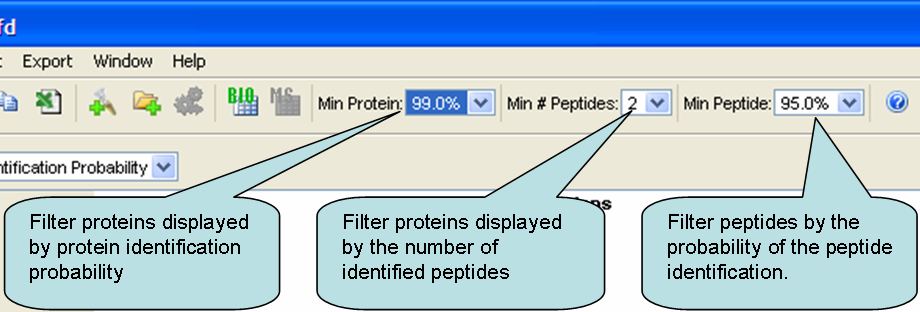Figure 1. Scaffold's display filters include Min Protein %, Min # of Peptides and Min Peptide %

Peptide Filter

Now you might ask, “If this is all there is to it, what is the purpose of the number-of-peptides filter?” Its purpose is to give you a backup method if you suspect the algorithm that calculates the protein probabilities.

The third filter, the filter on peptides, works with the number-of-peptides filter. Say for example, that you want to see proteins with two peptides detected. This only makes sense if you also specify the criteria for accepting these two peptides. To continue our example, your filter criteria could be two peptides each of which is at least 95% sure.

Assumption 1: Probabilities Displayed are Estimates of True Probabilities

The probabilities are calculated from a set of algorithms. These algorithms do not tell you how accurate they are. You have to remember that you should attribute limited precision to these estimates of the probabilities.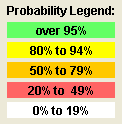Figure 2. Scaffold's probability legend

To remind you that the numbers displayed are surrogates for ranges of probabilities, Scaffold color codes all the probabilities. These color codes are meant to suggest that green probabilities are reasonably precise (±2), the yellow less so (±7), the orange still less(±10), and the red fairly imprecise (±15). These are only rough guidelines. As you will see in the following, how accurately the estimated probabilities approximate the real probabilities depends very strongly on the data set.

In the rest of this paper, we will discuss Scaffold’s three statistical graphs, how each shows you a window into one of Scaffold’s three main statistical algorithms, and how to use each to verify that Scaffold is working on a sound statistical footing. Then we will return to filters and look at your fall back position, that is, how you can analyze your data without using statistical probabilities.

Histograms And Peptide Probabilities

Scaffold has three graphs that show statistics about your data set, found on the Statistics page. Scaffold uses Bayesian statistics to estimate the peptide probabilities. The peptide probabilities are calculated by an algorithm developed by Keller, et. al. Anal. Chem. (2002) 74(20):5383. This algorithm is also used by PeptideProphet, a part of the Trans-Proteome Pipeline distributed by the Institute for Systems Biology. Let's look at the assumptions behind this algorithm and show data sets that don’t meet the assumptions. Later you will see what alternatives you have if the assumptions are not valid for your data set.

Assumption 2: The Data set has both correct and incorrect peptide spectrum matches

The first graph we will look at is the histogram which shows how the peptide probabilities are calculated. Each column in the histogram is a count of the number of spectra that matched to a peptide at the peptide score on the x-axis. In an ideal case all the correctly matched spectra will have high scores and all the incorrect matches will have low scores. For real data the correct and incorrect distributions usually overlap and the histogram will look like this: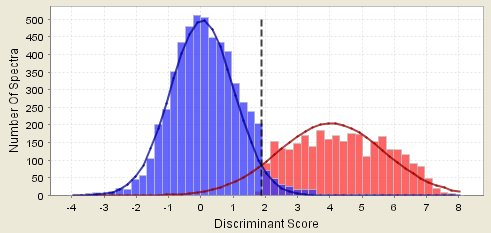Figure 3. A histogram showing a "good" distribution

Scaffold estimates peptide probabilities using the distribution of the incorrect matches to play much the same role as the distribution arrived at by searching a reversed database.

Counter example 2a. This assumption breaks down if all the peptide/spectrum matches are correct. We have seen this happen when a) the database searched contained only the one protein known to be in the sample and b) when the search engine (for example X! Tandem) is set to record only the correct matches and throw out the rest, or the bad spectra are filtered out before loading in Scaffold as in the histogram below.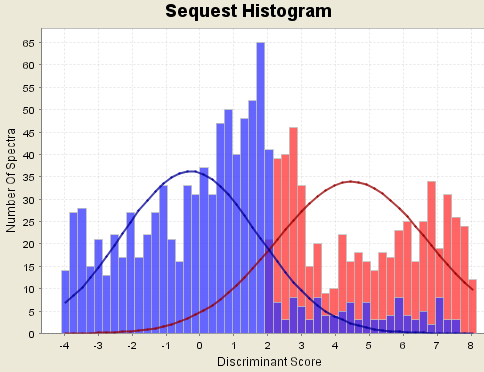Figure 4. Histogram showing bad curve fitting

Counter example 2b. This assumption breaks down if all the peptide/spectrum matches are incorrect. We have seen this happen when a) the wrong database was searched, and b) when a gel spot has no proteins with detectable peptides

Assumption 3: The data set has enough spectra to fit curves in the histogram

Scaffold fits two curves to this two hump histogram. The distribution on the left is taken to be the incorrect matches and the distribution on the right the correct matches. Discriminant scores are converted to probabilities by looking at the fitted curves rather than the original histograms. The Bayesian estimate of the peptide probability is the ratio at the search score observed for the peptide of the height of the correct distribution curve divided by the sum of the correct and incorrect distributions.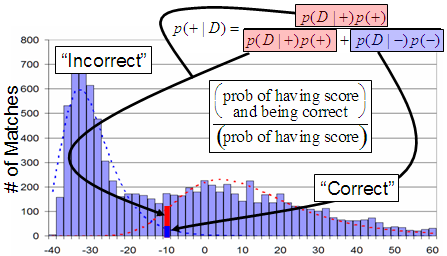Figure 5. Calculations in Scaffold's probability histogram

Counter example 3a. This assumption partially breaks down if the histograms are so very jagged that fitting smooth curves to them leads to inaccuracies. This is often the case when there are few spectra in the sample. In this case it would also be wise to assume the estimated peptide probabilities have large error bars associated with them where the probabilities where the distributions overlap. That is, the probabilities are only rough estimates. In fact this can easily happen if you ask Scaffold to search with X! Tandem on the subset of proteins that have already been found. Scaffold combats this by adding in random proteins to make the subset database large enough so the statistics will work for the X! Tandem results.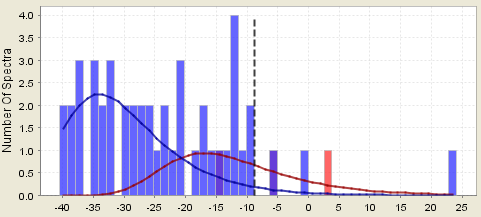Figure 6. A histogram showing too few spectra

Counter example 3b. If the database size is too small, then the discriminant scores may be biased. For SEQUEST, the discriminant score factors in deltaCn. If the database is very small, deltaCn tends to be way too big. Similarly for Mascot, the discriminant score factors in the Mascot Identity score. If the database is very small, the Identity score tends to be way too small. Any severe bias in the discriminant scores will adversely affect the accuracy of the peptide probabilities.

Assumption 4: The data set has enough correct matches so that two distributions can be fit to the histogram

Counter example 4a. This assumption breaks down if the tail of the incorrect distribution obscures the correct distribution. The incorrect distribution is fit to the large lump on the left of the histogram and its right-hand tail is estimated from the theoretical distribution. If the incorrect distribution is too much bigger than the correct distribution, then the error in fitting this tail or the error in the assumption of what type of curve fits the distribution may lead to inaccuracies. This situation occurs, for example, when an linear ion trap is set to trigger many MS/MS scans on noise.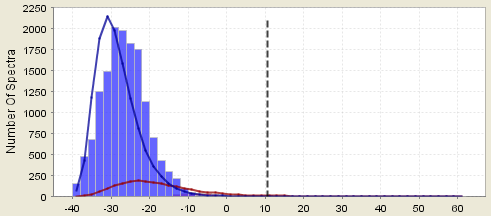Figure 7. Histogram showing good matches obscured by bad matches

Counter example 4b. This assumption partially breaks down if the correct distribution is very wide and flat. This sort of distribution makes it hard to accurately determine the center of the correct distribution. In this case the high probabilities are good estimates, but the low probabilities may be poor estimates.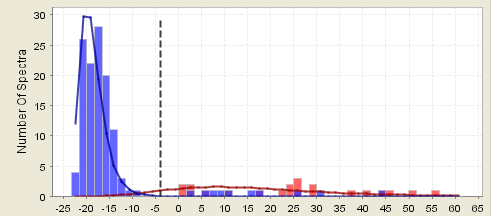Figure 8. A histogram showing a wide distribution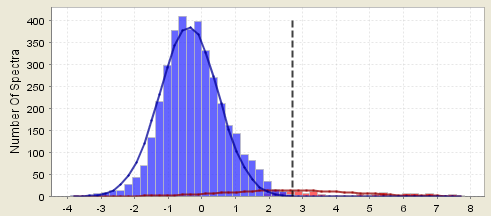Figure 9. A histogram showing a flat distribution

Assumption 5: Correct proteins will have peptides in the correct peptide distribution

Counter example 5. This assumption breaks down if there are many proteins identified by only one peptide and your filter criteria is that two peptides are required to positively identify a protein. In the following histogram peptides are color coded. Those belonging to proteins that passed the filter are colored red, the other peptides are blue, and the overlaps are purple. In the first figure, one peptide hits are accepted and show up red in the histogram. In the second figure, the same data is shown but now two peptides are required to call the protein. The blue peptides on the right are your one-hit wonders.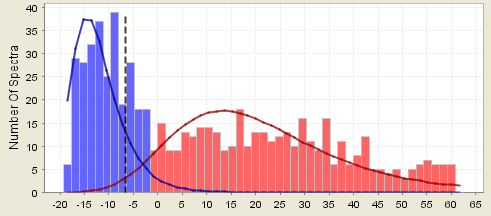Figure 10. A histogram where one hit wonder peptides are accepted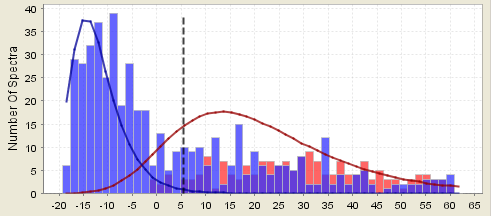Figure 11. Distribution shown above with two hit proteins accepted

From looking at how these two distributions overlap you can get an idea of how much precision you should attribute to the estimates of the probabilities. The very high scoring peptides are quite likely in the correct camp. That is, the estimates of their probability are pretty good. The peptides in the regions where the distribution curves overlap are much more susceptible to vagaries in the data.

Assumption 6: The best approximation for each peptide is learned from the distribution of all peptides

In Mascot, SEQUEST, X! Tandem and most other peptide scoring schemes peptides above a threshold are taken as good, irrespective of the characteristics of the peptide. Scaffold recognizes that this threshold assumption is dubious. A more defensible assumption is that all the peptides in a particular sample, processed in the same way, and searched against the same database have similar characteristics. The peptide probability algorithm learns from the distribution of scores for all peptides in each sample what a good peptide match looks like and what a poor peptide match looks like. For example, peptides in a cell lysate may have different characteristics that those in a serum sample and Scaffold adjusts the probabilities accordingly.

Counter example 6. The spreadsheet histogram below of discriminate scores for two distinctly different peptides shows that there isn't one distribution that accurately represents both peptides, or even the FYTVISSLK peptides with charge 1+ and charge 2+. That is, the distribution of correct scores depends on characteristics of the peptide. In other words, averaging all the peptides in the sample as Scaffold does is only an approximation.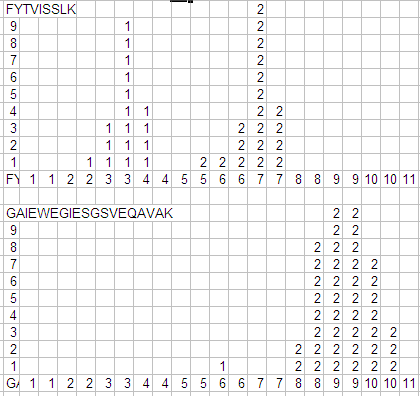Figure 12. Histogram reflecting very different distributions

Unfortunately, there is no way to quantify this problem directly in Scaffold. However, Scaffold tries to minimize it by carefully selecting a discriminate score. SEQUEST has several scores (XCorr, deltaCn, RankSP, deltaMass). The discriminant score for SEQUEST is the combination of these scores which best separates the correct from the incorrect matches. Although Mascot has different scores (Ion Score and Identity Score), the same principle applies: the discriminant score is chosen to best separate the good matches from the bad.

Scaffold is Conservative

Scaffold doesn't completely trust the peptide algorithm. To remain on the conservative side, the largest peptide probability that Scaffold assigns, no matter what the algorithm says, is 95%.

If Assumptions Fail

If you believe one or more of these assumptions fail for your data set, it's best not to believe the calculated peptide probabilities. Fortunately Scaffold provides a fall back. You can create custom filters based directly on the SEQUEST or Mascot scores. To create a custom filter, click "Custom" on the "Min Peptide" filter to bring up a dialog box.
You can now filter on the number of peptides that match your custom filter.

Scatterplots And Combining Multiple Search Engines

The previous section showed how by examining histograms you can check the assumptions that Scaffold uses for converting search engine scores into peptide probabilities. But the search engines themselves (Sequest, Mascot and so on) have built in hidden assumptions about what makes a good peptide score. Since different search engines make different assumptions, they score peptides differently. You can take advantage of this fact with Scaffold.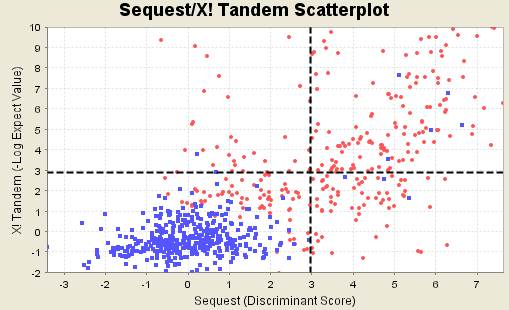Figure 13. Scatterplot of good and bad matches when SEQUEST and X! Tandem are run

If you have searched your MS/MS data set with more than one search engine, say SEQUEST and X! Tandem, Scaffold combines these searches to calculate the peptide probability. The following figure shows that the histograms are related to the scatter plots. The histogram on top shows the counts of scores in the scatter plot directly beneath each bin. Similarly the histogram on the side shows the counts of scatter plot scores taken as rows.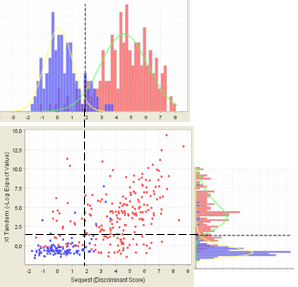Figure 14. A scatterplot and its related histograms

By examining the scatter plots on the Statistics page, you can determine if the assumptions that Scaffold makes about joining search engine results are reasonable.

Assumption 7: Searching with several search engines will find more peptides

Different search engines make different assumptions about what makes a good peptide score. For example, Sequest uses an correlation function that is pretty good at searching spectra that are obscured by noise, whereas Mascot uses a more sophisticated method of separating random hits from true ones for large databases. From this it follows that each search engine identifies certain peptides well, others not so well. Most of the data we have looked at shows a surprising amount of difference between search engines.

Counter example 7a. The following data set is highly unusual because it shows two search engines whose scores are highly correlated. Doing both searches is largely a waste of time.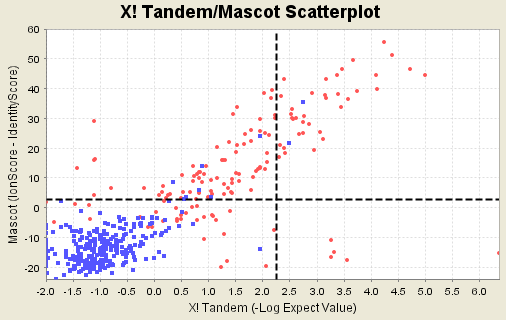Figure 15. Histogram showing strong correlation between search engine results

Counter example 7b. If one search engine searches the wrong database, the scores for that search engine will not contribute anything worthwhile.

Assumption 8: When different search engines agree the peptide identified is more likely to be valid

This too seems like common sense based on the same observation that different search engines make different assumptions.

Counter example 8. Since all search engines examine the same peaks in the same spectra, they can make the same error.

If Assumptions Fail

If you find searching with multiple data sets isn't providing better results on your data sets, then obviously it makes sense to use only your favorite search engine.

Protein Probabilities Derived From Peptides

Scaffold calculates protein probabilities by combining the probabilities of all the peptides in the protein. The algorithm that calculates protein probabilities was invented by Nesviszhskii, et. al., Anal Chem 2003 Sep 1;75(17):4646-58. The same algorithm is used by ProteinProphet a part of the Trans Proteome Pipeline distributed by the Institute for Systems Biology.

Scaffold provides a graph on its Statistics page that shows for each sample how the peptide probabilities are combined to estimate the protein probability. In this graph the red line connecting squares shows the calculated protein probability is for a single peptide hit. The blue line shows the calculated protein probability if two peptides are detected. For simplicity the graph shows only the line where both peptides have the same probability. For example, if you have two peptides at 75%, the protein probability is 92%. The other lines on the graph are for 3, 4 and 5 peptides again assuming, all peptides have the same probability.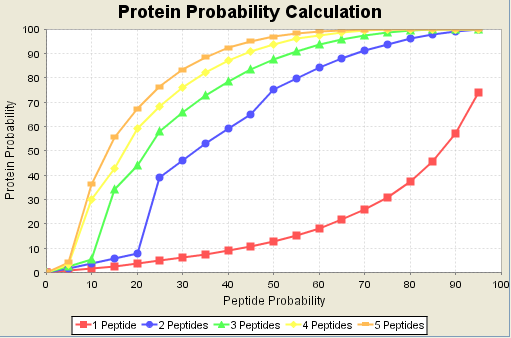Figure 16. Protein probabilities are effected by the number of peptides required

Assumption 9: Protein probability is accurate because peptide probabilities are.

The original protein probability algorithm uses the peptide probabilities, even if these probabilities are low. This allows a more complete use of your data set and it can discover protein hits that would otherwise be missed. Statistically this algorithm is valid if the peptide probabilities are accurate. However, as we saw under Assumption 8 above the estimates of the peptide probabilities have uncertainties and at lower probabilities these uncertainties can be fairly large. The protein probability algorithm doesn't take these uncertainties into account.

Counter example 9. A data set with the characteristics shown in the graph above will be assigned a protein probability of near 100% if it has 5 peptides at 60% probability. It may well be that if you visually inspect these 5 peptides, you won't believe a single one. Many people won't accept a peptide with that low a score, but the algorithm does. Beware of Garbage In, Garbage Out.

Scaffold is Conservative

Scaffold has modified the protein probability algorithm to consider only those peptides which are labeled good. By default, peptides with a probability greater than 50% are considered good, and those with probability less than 50% are not. Scaffold is conservative in this regard because we found cases where very poor quality spectra were affecting the protein probability unreasonably.

If this Assumption Fails

You can review the peptides that contribute to the protein score by looking at the checkbox in the "Valid" column in Scaffold's peptides list. You can check or uncheck the checkbox based upon your own evaluation of the quality of the match between spectrum and peptide. If you do this, bear in mind that if there are multiple spectra matching a peptide, the protein probability only depends upon the largest good (that is "checked") peptide probability. Multiple spectra matching the same peptide do not effect the protein probability.

Assumption 10: The importance of multiple peptides hits depends on their prevalence in the data set.

The protein probability algorithm is a learning algorithm. It learns by looking at all the proteins identified in each data set. It figures that good proteins should look like other good proteins in terms of how many peptides they have been identified with. If most peptides are one-hit wonders, it treats one-hit wonders well. If many proteins are identified by multiple peptides, it down-grades one-hit wonders. This learning mode means that Scaffold optimizes itself for a wide variety of samples and preparation techniques.

Counter example 10a. Many people have found from experience to not trust single peptide hits, no matter what. The protein probability algorithm may even make this worse since it will upgrade the protein probability of what looks like a one-hit wonder if it is supported by one or two very low probability peptides.

Counter example 10b. Many times people want to see one-hit wonders. If the sample has a number of high abundance large proteins with many peptides identified in these proteins, the protein probability algorithm may make it very hard to observe one-peptide hits. For example, in the data set below, the red line shows that no matter how good the peptide match is, the protein probability will be no better than about 25%.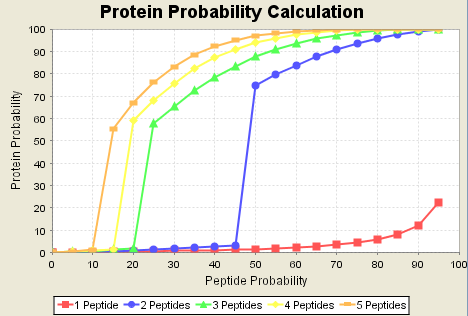Figure 17. Note one hit protein probability is never greater than 25%

If this Assumption Fails

If you don't believe the protein probabilities, Scaffold lets you filter your data based upon the number of peptides and your criteria for believing a peptide hit. To eliminate one-hit wonders, set the number of peptides filter to 2. To see one-hit wonders, set the protein probability to 20% and the number of peptides filter to 1.

Conclusion

Talk to any statistician. They will caution you about believing any conclusions when you have identifications for hundreds of proteins and only a few samples. Coincidences do happen. If you load enough data, some unlikely events are almost sure to happen. Your statistician will probably tell you that your best defense is to run lots of samples. To encourage you to do this, Scaffold lets you compare multiple samples.

If you deal with people who push statistics at you, you should read the book “How to Lie with Statistics” by Darrell Huff. It shows you how to defend yourself from people who like to lie with statistics.

In Conclusion

Don't be fooled by your proteomics software. Learn if its assumptions are true for your data set. This applies to all software, not just Scaffold. But with Scaffold you can explicitly check its assumptions. If you check and your data set satisfies the Scaffold assumptions, then you can trust the results. If some of the assumptions don't hold Scaffold generally gives you a fall back option.

Was this article helpful?
0 out of 0 found this helpful
Have more questions? Submit a request

#### 0 Comments

Please sign in to leave a comment.